# Class 10 Maths NCERT solutions for Surface Area and Volume Exercise 13.1

In this page we have Class 10 Maths NCERT solutions for Surface Area and Volume for Exercise 13.1 on pages 244,245. Hope you like them and do not forget to like , social_share and comment at the end of the page.

This exercise is based on the surface area of an object formed by combining any two of the basic solids, namely, cuboid, cone, cylinder, sphere and hemisphere
Formula to used
The total surface area of the any new solid is the sum of the curved surface areas of each of the individual parts.
TSA of new solid = CSA of One part + CSA of Second part + CSA of Third part
where TSA, CSA stand for ‘Total Surface Area’ and ‘Curved Surface Area’ respectively.
Unless stated otherwise, take $\pi = \frac {22}{7}$.

## Surface Area and Volume Exercise 13.1

Question 1.
2 cubes each of volume 64 cm3are joined end to end. Find the surface area of the resulting cuboid.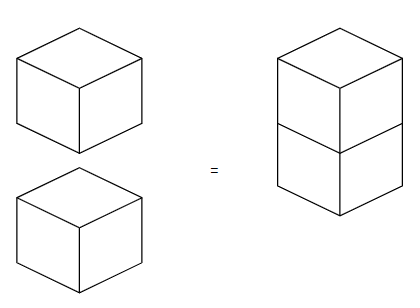Volume of each cube(a3) = 64 cm3
a3= 64 cm3
So a = 4 cm
Side of the cube = 4 cm
Length of the resulting cuboid = 4 cm
Breadth of the resulting cuboid = 4 cm
Height of the resulting cuboid = 8 cm
∴ Surface area of the cuboid = 2(lb + bh + lh)
= 2(8×4 + 4×4 + 4×8)cm2
= 2(32 + 16 + 32) cm2
= (2 × 80) cm2= 160 cm2

Question 2.
A vessel is in the form of a hollow hemisphere mounted by a hollow cylinder. The diameter of the hemisphere is 14 cm and the total height of the vessel is 13 cm. Find the inner surface area of the vessel.
Diameter of the hemisphere = 14 cm
Radius of the hemisphere(r) = 7 cm
Height of the cylinder(h) = 13 - 7 = 6 cm
Also, radius of the hollow hemisphere = 7 cm
Inner surface area of the vessel = CSA of the cylindrical part + CSA of hemispherical part
$= (2\ pi rh + 2 \pi r^2)= 2 \pi r(h+r)$
$= 2 \times \frac {22}{7} \times 7 (6+7)= 572$ cm2

Question 3.
A toy is in the form of a cone of radius 3.5 cm mounted on a hemisphere of same radius. The total height of the toy is 15.5 cm. Find the total surface area of the toy.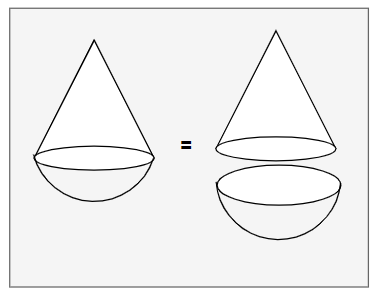Radius of the cone and the hemisphere(r) = 3.5 = 7/2 cm
Total height of the toy = 15.5 cm
Height of the cone(h) = 15.5 - 3.5 = 12 cm
Now Slant height of the cone is given by
L=√(h2 + r2)
=√(122 + 3.52)
=12.5
Curved Surface Area of cone = πrl = 22/7 × 3.5 ×12.5 = 137.5 cm2
Curved Surface Area of hemisphere = 2πr2= 2 × 22/7 × (3.5)2= 77 cm2
Total surface area of the toy = CSA of cone + CSA of hemisphere = (137.5+ 77) cm2
= 214.5cm2
The total surface area of the toy is 214.5cm2

Question 4.
A cubical block of side 7 cm is surmounted by a hemisphere. What is the greatest diameter the hemisphere can have? Find the surface area of the solid.
Each side of cube = 7 cm
Therefore Radius of the hemisphere = 7/2 =3.5 cm
Total surface area of solid = Surface area of cubical block + CSA of hemisphere - Area of base of hemisphere
Total surface area of solid = 6×(side)2+ 2πr2-πr2= 6×(side)2+ πr2= 6×(7)2+ (22/7 × 3.5 × 3.5)
= 332.5 cm2
The surface area of the solid is 332.5 cm2

Question 5.
A hemispherical depression is cut out from one face of a cubical wooden block such that the diameter l of the hemisphere is equal to the edge of the cube. Determine the surface area of the remaining solid.
Diameter of hemisphere = Edge of cube = l
Total surface area of solid = Surface area of cube + CSA of hemisphere - Area of base of hemisphere
TSA of remaining solid = 6 (edge)2+ 2πr2- πr2= 6l2+ πr2
= 6l2+ π(l/2)2= 6l2+ πl2/4= l2/4(24+ π) sq units

Question 6.
A medicine capsule is in the shape of a cylinder with two hemispheres stuck to each of its ends. The length of the entire capsule is 14 mm and the diameter of the capsule is 5 mm. Find its surface area.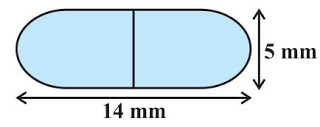It can be observed from the figure that there are two hemispherical parts with radius = 5/2 = 2.5 mm
Also, there is one cylinder whose radius is equal to the hemisphere and length of the cylinder is (14 - 5) = 9 mm.
CSA area of the cylinder= 2πrh=2π×2.5×9=45 π cm2
CSA area of two hemisphere=4 πr2 =25 π cm2
Total Surface Area
=45 π+25 π
=220 cm2

Question 7
A tent is in the shape of a cylinder surmounted by a conical top. If the height and diameter of the cylindrical part are 2.1 m and 4 m respectively, and the slant height of the top is 2.8 m, find the area of the canvas used for making the tent. Also, find the cost of the canvas of the tent at the rate of Rs 500 per m2. (Note that the base of the tent will not be covered with canvas.)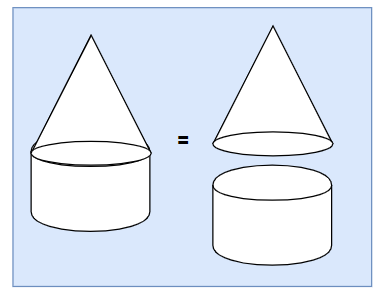Radius of cylinder = 2 m, height = 2.1 m and slant height of conical top = 2.8 m
Curved Surface Area of cylindrical portion
=2πrh=2π×2×2.1=8.4 π m2
Curved Surface Area of conical portion
=πrl= 5.6 π m2
Total Curved Surface Area
=CSA for Cylinder + CSA for Cone
=44m2
Now Cost of canvas = Rate x Surface Area
= 500 x 44 = Rs. 22000

Question 8
From a solid cylinder whose height is 2.4 cm and diameter 1.4 cm, a conical cavity of the same height and same diameter is hollowed out. Find the total surface area of the remaining solid to the nearest cm2.
Radius = 0.7 cm and height = 2.4 cm.
Slant Height of the cone can be calculated as
L=√(h2 + r2)
=√(2.42 + .72)
=2.5cm
Total Surface Area of Structure = Curved Surface Area of Cylinder + Area of top of cylinder + Curved Surface Area of Cone
Curved Surface Area of Cylinder=2 πrh=2 π×.7×2,4= 10.56 cm2
Area of top of the cylinder=πr2 =1.54 cm2
Curved Surface Area of Cone =πrl =5.5 cm2
Hence, remaining surface area of structure
= Curved Surface Area of Cylinder + Area of top of cylinder + Curved Surface Area of Cone
=10.56+1.54+5.5=17.6 cm2 =18 cm2 (approx.)

Question 9
A wooden article was made by scooping out a hemisphere from each end of a solid cylinder, as shown in figure. If the height of the cylinder is 10 cm, and its base is of radius 3.5 cm, find the total surface area of the article.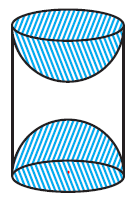Total Surface Area of Structure = CSA of Cylinder + CSA of two hemispheres
Curved Surface Area of Cylinder =2 πrh=2 π×3.5×10=220 cm2
Surface Area of Sphere =4 πr2 =154 cm2
Total Surface Area
=220 cm2 + 154 cm2
=374cm2

## Summary

1. Class 10 Maths NCERT solutions for Surface Area and Volume Exercise 13.1 has been prepared by Expert with utmost care. If you find any mistake.Please do provide feedback on mail.You can download this as pdf
2. This chapter 13 has total 5 Exercise 13.1 ,13.2,13.3 ,13.4 and 13.5. This is the First exercise in the chapter.You can explore previous exercise of this chapter by clicking the link below

• Notes
• Assignments
• NCERT Solutions
• NCERT Solution Surface Area and Volume Class10 Exercise 13.1
• NCERT Solution Surface Area and Volume Class10 Exercise 13.2
• NCERT Solution Surface Area and Volume Class10 Exercise 13.3Go back to Class 10 Main Page using below links

### Practice Question

Question 1 What is $1 - \sqrt {3}$ ?
A) Non terminating repeating
B) Non terminating non repeating
C) Terminating
D) None of the above
Question 2 The volume of the largest right circular cone that can be cut out from a cube of edge 4.2 cm is?
A) 19.4 cm3
B) 12 cm3
C) 78.6 cm3
D) 58.2 cm3
Question 3 The sum of the first three terms of an AP is 33. If the product of the first and the third term exceeds the second term by 29, the AP is ?
A) 2 ,21,11
B) 1,10,19
C) -1 ,8,17
D) 2 ,11,20